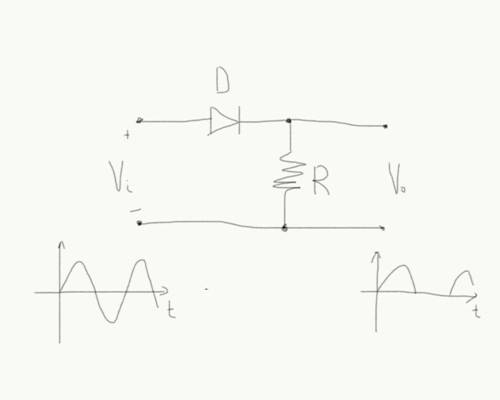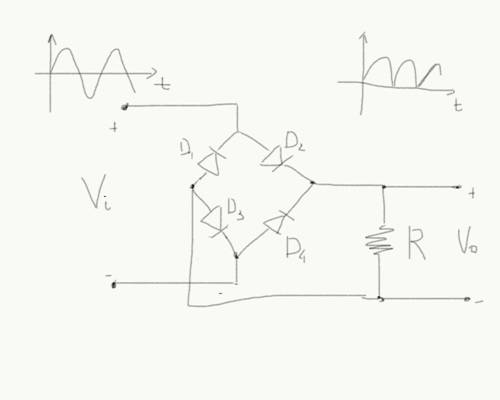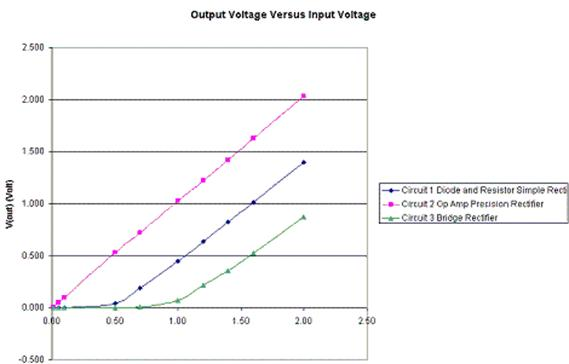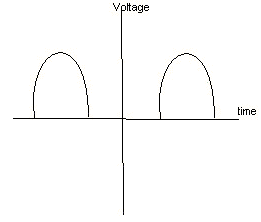Peter Lau

Bill Chun Wai Hung

Lab 9 Report ¨C Voltage Rectifier

26 July 2004

A. Describe the Set-up

There are 3 circuits in this lab, and each circuit has part I and Part II.
The Circuit 1 setup is shown in figure 1.Figure 1

The Circuit 2 setup is shown in figure 2.Figure 2

The Circuit 3 setup is shown in figure 3.Figure 3

B. Describe Inputs

Resistor: The Resistor used is 1k ohm

Diode: The model for the diodes are D1N4002
Op Amp: model LM4862N

Stimuli:

For Part I,

The +5V and -5V voltage input is connected across the input of the circuit (Vi). The output voltage (Vo) is measured by the DVM.

For Part II,

The AC function generator produce a sine curve input voltage across the input of the circuit (Vi). The output voltage (Vo) is measured by the oscilloscope.

C. Describe What you Observe

Part I.

Circuit 1 Simple Rectificer

The result of V(in) (=Vi in the prelab) versus V(out) (=Vo in the prelab) is shown in Table 1 and Figure 4 and Figure 5.
Table 1.

 V(in) (Volt) V(out) (Volt) 0.01 0.000 0.05 0.000 0.10 0.000 0.50 0.044 0.70 0.188 1.00 0.450 1.20 0.636 1.40 0.823 1.60 1.014 2.00 1.399

Part I Circuit 2 Precision Rectificer.

The result of V(in) (=Vi in the prelab) versus V(out) (=Vo in the prelab) is shown in Table 2 and Figure 4 and Figure 5.
Table 2.

 V(in) (Volt) V(out) (Volt) 0.01 0.012 0.05 0.052 0.10 0.102 0.50 0.531 0.70 0.722 1.00 1.031 1.20 1.222 1.40 1.422 1.60 1.625 2.00 2.031

Part I Circuit 3 Bridge Rectificer.

The result of V(in) (=Vi in the prelab) versus V(out) (=Vo in the prelab) is shown in Table 3 and Figure 4 and Figure 5.
Table 3.

 V(in) (Volt) V(out) (Volt) 0.01 0.003 0.05 0.003 0.10 0.003 0.50 0.003 0.70 0.006 1.00 0.075 1.20 0.218 1.40 0.360 1.60 0.523 2.00 0.875Figure 4

Part II.
Circuit 1Figure 5. Circuit I and Circuit II Oscilloscope outputFigure 6. Circuit III Oscilloscope output

Circuit I. V(peak to peak) = 2V, the V(out peak voltage) = 0.435V

Circuit II. V(peak to peak) = 2V, the V(out peak voltage) = 1.062V

Circuit III. V(peak to peak) = 6V, the V(out peak voltage) = 1.766V

D. What you can deduce

Q1) What is the difference between the half wave rectifier and the precision rectifier?

The half wave rectifier truncates one half of the sine wave plus an extra 0.6V, owing to the fixed voltage drop across the diode. The full wave rectifier circuit does not exhibit this behavior.

Q2) Why is the threshold of the precision rectifier lower than the threshold of the simple rectifier?

The output is connected to the inverting input, while the input is fed into the non-inverting input. The two inputs of an opamp are held at the same voltage by the feedback loop. This is why the output tracks the input precisely (without a voltage drop) when the diode is forward biased. Current cannot flow at all when the diode is reverse biased, so the feedback loop is broken and the input is blocked and not reflected in the output.

Q3) What is the minimum signal needed at the input of the diodes bridge in order to be able to see an output

The experimental minimum signal needed at the input of the diodes is 1 voltage. The minimum voltage input is shown in Figure 5 with the lowest curve on the plot.

The experimental minimum input voltage agrees with the expected is minimum input voltage = 0.5 x 2 = 1V.  The 0.5V is the cutoff voltage of each diodes, and there are two active diodes acting on the current in the circuit, so the minimum input voltage is 1V.

What I am planning to do in order to claim my circuit works.

Part I Circuit 1. The middle curve in figure 4 with minimum input voltage of 0.5V indicates one active diode in the circuit.

Part I Circuit 2. The top curve in figure 4 with minimum input voltage of almost 0V indicates the op amp and diode pair acting as a perfect diode.

Part I Circuit 3. The bottom curve in figure 4 with minimum input voltage of 1V indicates two active diode in the circuit.

Part II Circuit 1. The output waveform is like the one in Figure 5

Part II Circuit 2. The output waveform is like the one in Figure 5

Part II Circuit 3. The output waveform is like the one in Figure 6